Gauge your potential with Aakash BYJU'S JEE Main'23 Mock Test Gauge your potential with Aakash BYJU'S JEE Main'23 Mock Test

# JEE Main 2021 March 17 – Shift 1 Maths Question Paper with Solutions

JEE Main 2021 shift 1 Maths solutions along with the answer key is available at BYJU’S. JEE Main 2021 question paper for Maths (March Shift 1) is solved by subject experts in a detailed manner. Solving these questions will help students to improve their speed and accuracy for the upcoming exams. Students can download and solve the JEE Main 2021 shift 1 Maths question paper from the link mentioned below.

### JEE Main 2021 March 17th Shift 1 Maths Question Paper

Section A

1. a. y (1) = 1
2. b. y (1) = e1/2 - 1
3. c. y (1) = e1/2 - e-1/2
4. d. y (1) = e - 1

Solution:

dy / dx = (x - 1) y + (x - 1)

dy / dx = (x - 1) (y + 1)

(dy / (y + 1)) = (x - 1) dx

ln (y + 1) = (x2 / 2) - x + c

x = 0, y = 0 ⇒ c = 0

ln (y + 1) = (x2 / 2) - x

Putting x = 1, ln (y + 1) = (1 / 2) - 1 = - 1 / 2

y + 1 = e

y = e - 1

y (1) = e - 1

Question 2: The system of equations kx + y + z = 1, x + ky + z = k and x + y + zk = k2 has no solution if k is equal to

1. a. - 2
2. b. - 1
3. c. 1
4. d. 0

Solution:

$$D=\begin{vmatrix} k &1 &1 \\ 1&k &1 \\ 1&1 &k \end{vmatrix}=0$$

⇒ k(k2 – 1) – (k – 1) + (1 – k) = 0

⇒ (k – 1) (k2 + k – 1 – 1) = 0

⇒ (k – 1) (k2 + k – 2) = 0

⇒ (k –1) (k –1) (k + 2) = 0

⇒k = 1, k = 2

For k = 1 equation identical so k = –2 for no solution.

Question 3: The value of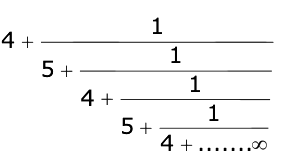is:

1. a. 2 + (4 / √5) (√30)
2. b. 4 + (4 / √5) (√30)
3. c. 2 + (2 / 5) (√30)
4. d. 5 + (2 / 5) √30

Solution:

y = 4 + 1 / [5 + (1 / y)]

⇒ y = 4 + y / [5y + 1]

⇒ 5y2-20y-4 = 0

⇒ y = [20 ± √400 + 80] / 10

⇒ y = [20 ± 4√30] / 10

y = [10 + 2√30] / 5

Question 4: If the Boolean expression (p ⇒ q) ⇔ (q * (~ P)) is a tautology, then the Boolean expression p * (~q) is equivalent to:

1. a. p ⇒ ~ q
2. b. p ⇒ q
3. c. q ⇒ p
4. d. ~q ⇒ p

Solution:

(p → q) ⇔ (q * ~ P)

 p q p → q q * ~ P ~ q ~ q ∧ p ~ (~ q ∧ p) T T T T F F T T F F F T T F F T T T F F T F F T T T F T

∴ ~ (~ q ∧ p) = q ∨ ~ p = ~ p ∨ q

∴ * is equivalent to ∨

∴ p * ~ q = p ∨~ q

= ~ q ∨ p

= q p

Question 5: Choose the incorrect statement about the two circles whose equations are given below:

x2 + y2 - 10x - 10y + 41 = 0 and x2 + y2 - 16x - 10y + 80 = 0

1. a. Distance between two centres is the average radii of both the circles
2. b. Circles have two intersection points
3. c. Both circles’ centres lie inside the region of one another
4. d. Both circles pass through the centre of each other

Solution:

C1 (5, 5), C2 (8, 5)

The position of C1(5, 5) in S2 = 0

= 25 + 25 – 80 – 50 + 80

= 0

The position of C2(8, 5) in S1 = 0

= 64 + 25 – 80 – 50 + 41

= 0

Question 6: The sum of possible values of x for tan-1 (x + 1) + cot-1 [1 / (x - 1)] = tan-1 (8 / 31) is:

1. a. - 33 /4
2. b. - 32 / 4
3. c. - 31 / 4
4. d. - 30 / 4

Solution:

Taking tan both sides

[(1 + x) + (x - 1)] / [1 - (1 + x) (x - 1)] = 8 / 31

[2x / (2 - x2)] = 8 / 31

4x2 + 31x - 8 = 0

x = - 8, 1 / 4

But at x = 1 / 4

LHS > π / 2 and RHS < π / 2

So, the only solution is x = – 8.

Question 7: Let

$$\vec{a}=2i-3j+4k,\vec{b}=7i+j-6k$$
. If
$$\vec{r}\times\vec{a}=\vec{r}\times\vec{b},\vec{r}.(i+2j+k)=-3,$$
, then
$$\vec{r}.(2i-3j+k)=$$
is equal to

1. a. 10
2. b. 13
3. c. 12
4. d. 8

Solution:

Question 8: The equation of the plane which contains the y-axis and passes through the point (1, 2, 3) is:

1. a. 3x + z = 6
2. b. 3x − z = 0
3. c. x + 3z = 10
4. d. x + 3z = 0

Solution:

Let the equation of the plane is a(x – 1) + b(y – 2) + c (z – 3) = 0

Y–axis lies on it D.R.’s of y - axis are 0, 1, 0

0.a + 1.b + 0.c = 0 ⇒ b = 0

Equation of plane is a(x – 1) + c (z – 3) = 0

x = 0, z = 0 also satisfy it –a – 3c = 0 ⇒ a = –3c

–3c (x – 1) + c (z – 3) = 0

–3x + 3 + z – 3 = 0

3x – z = 0

Question 9: If A =

$$\begin{pmatrix} 0 &sin\alpha \\ sin\alpha &0 \end{pmatrix}$$
and det (A2 - (1 / 2) I) = 0, then a possible value of ɑ is:

1. a. π / 6
2. b. π / 2
3. c. π / 3
4. d. π / 4

Solution:

Question 10: The line 2x - y + 1 = 0 is a tangent to the circle at the point (2, 5) and the centre of the circle lies on x - 2y = 4. Then, the radius of the circle is:

1. a. 4√5
2. b. 3√5
3. c. 5√3
4. d. 5√4

Solution: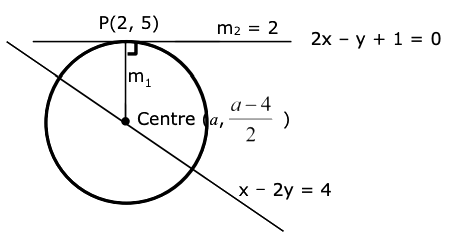m1 * m2 = – 1

[{[a - 4] / 2}-5 / (a - 2)] * 2 = - 1

[a - 14] / [a - 2] = - 1

a– 14 = 2 – a

2a = 16

a = 8

Centre (8, 2)

= √45

= 3√5

Question 11: Team ‘A’ consists of 7 boys and n girls and Team ‘B’ has 4 boys and 6 girls. If a total of 52 single matches can be arranged between these two teams when a boy plays against a boy and a girl plays against a girl, then n is equal to

1. a. 5
2. b. 6
3. c. 2
4. d. 4

Solution:

7 × 4 + 6 × n = 52

6n = 24

n = 4

Question 12: In a triangle PQR, the coordinates of the points P and Q are (-2, 4) and (4, -2) respectively. If the equation of the perpendicular bisector of PR is 2x – y + 2 = 0, then the centre of the circumcircle of the △PQR is:

1. a. (-2, -2)
2. b. (0, 2)
3. c. (-1, 0)
4. d. (1, 4)

Solution: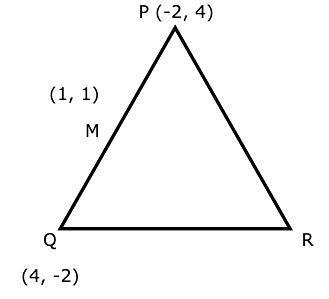Perpendicular bisector of PR : 2x – y + 2 = 0 .....(1)

Midpoint of PQ is M (1, 1).

Equation of perpendicular bisector of PQ : x – y = 0 ......(2)

POI of equation (1) & (2) is circumcentre.

So, circumcentre is (–2,–2).

Question 13: If cot-1 (ɑ) = cot-1 2 + cot-1 8 + cot-1 18 + cot-1 32 + …….. upto 100 terms, then ɑ is:

1. a. 1.03
2. b. 1.00
3. c. 1.01
4. d. 1.02

Solution:

RHS = ∑n=1100 cot-1 2n2 = ∑n=1100 tan-1 (2 / 4n2)

= ∑n=1100 tan-1 {[(2n + 1) - (2n - 1)] / [1 + (2n + 1) (2n - 1)]}

= ∑n=1100 tan-1 (2n + 1) - tan-1 (2n - 1)

= tan-1 201 – tan-11

= tan-1 (200 / 202)

cot-1 ɑ = cot-1 [101 / 100]

ɑ = 1.01

Question 14: Which of the following statements is incorrect for the function g (ɑ) for ɑ ∈ R such that g (ɑ) = ∫π/6π/3 sinɑ x / [cosɑ x + sinɑ x] dx

1. a. g (ɑ) is a strictly decreasing function
2. b. g (ɑ) has an inflexion point at ɑ = - 1/2
3. c. g(ɑ) is an even function
4. d. g(ɑ) is a strictly increasing function

Solution:

1. Answer: (a OR b OR c or Bonus)

g (x) = π/12 by applying prop. x → a + b – x.

Question 15: If the fourth term in the expansion of (x + xlog_{2} x)7 is 4480, then the value of x where x ∈ N is equal to:

1. a. 4
2. b. 3
3. c. 2
4. d. 1

Solution:

7C3 x4 (xlog2 x)3 = 4480

35x4 (xlog2 x)3 = 4480

x4 (xlog2 x)3 = 128

Take log w.r.t base 2 we get 4 log2 x + 3 log2 (xlog2 x) = log2 128

Let log2 x = y

4y + 3y2 = 7

y = 1, - 7 / 3

log2 x = 1, - 7 / 3

x = 2, x = 2–7/3

Question 16: Two dice are rolled. If both dices have six faces numbered 1, 2, 3, 5, 7 and 11, then the probability that the sum of the numbers on the top faces is less than or equal to 8 is:

1. a. 17 / 36
2. b. 4 / 9
3. c. 5 / 12
4. d. 1/2

Solution:

n (S) = 36

The possible ordered pairs are (1, 1), (1, 2), (1, 3), (1, 5), (1, 7), (2, 1), (2, 2), (2, 3), (2, 5), (3, 1), (3, 2), (3, 3), (3, 5), (5, 1), (5, 2), (5, 3), (7, 1)

Number of ordered pair = 17

Probability = 17 / 36

Question 17: The inverse of y = 5logx is:

1. a. x = 5logy
2. b. x= ylog5
3. c. x = y1/log5
4. d. x = 51/logy

Solution:

y = 5logx

log5 y = loge x

x = elog5y

Question 18: In a school, there are three types of games to be played. Some of the students play two types of games, but none play all three games. Which Venn diagrams can justify the above statements.1. a. P and R
2. b. P and Q
3. c. None of these
4. d. Q and R

Solution:

In are the (A), (B), (C) there are some students which play all three games hence no Venn diagram is correct.

Question 19: The area of the triangle with vertices A (z), B (iz) and C (z + iz) is:

1. a. (1 / 2) |z + iz|2
2. b. 1
3. c. 1 / 2
4. d. (1 / 2) |z|2

Solution: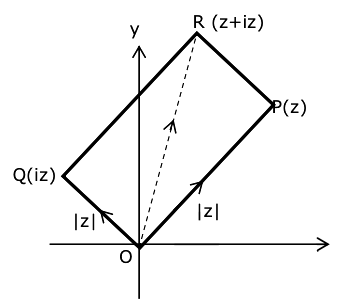Area of triangle = (1 / 2) (area of square) = (1 / 2) |z|2

Question 20: The value of limx→0+ [cos-1 (x - [x]2) . sin-1 (x - [x]2)] / [x - x3], where [x] denotes the greatest integer ≤ x is:

1. a. 0
2. b. π / 4
3. c. π / 2
4. d. π

Solution:

limx→0+ [cos-1 (x - [x]2) . sin-1 (x - [x]2)] / [x - x3]

limx→0+ [cos-1 (x - [x]2) . sin-1 (x - [x]2)] / [x (1 - x2)]

limx→0+ [cos-1 x sin-1 x] / x = π / 2

Section B

Question 21: Let there be three independent events E1, E2 and E3. The probability that only E1 occurs is ɑ, only E2 occurs is β and only E3 occurs is 𝛾. Let ‘p’ denote the probability of none of the events that occur that satisfies the equations (ɑ - 2β) p = ɑβ and (β - 3𝛾) p = 2β𝛾. All the given probabilities are assumed to lie in the interval (0, 1) Then, [probability of occurrence of E1] / [probability of occurrence of E3] is equal to _______

Solution:

Let x, y, z be probability of B1, B2, B3 respectively.

⇒ x(1 – y)(1 – z) = ɑ

⇒ y(1 – x)(1 – z) = β

⇒ z(1 – x)(1 – y) = 𝛾

⇒ (1 – x) (1 – y) (1 – z) = ⍴

Putting in the given relation we get x = 2y and y = 3z

⇒ x = 6z

⇒ x / z = 6

Question 22: If the equation of the plane passing through the line of intersection of the planes 2x - 7y + 4z - 3 = 0, 3x - 5y + 4z + 11 = 0 and the point (-2, 1, 3) is ax + by + cz - 7 = 0, then the value of 2a + b + c - 7 is __________

Solution:

The equation of plane can be written using the family of planes: P1 + λP2 = 0.

(2x –7y + 4z – 3) + λ (3x – 5y + 4z + 11) = 0

It passes through (-2, 1, 3).

(- 4 - 7 + 12 - 3) + λ (- 6 - 5 + 12 + 11) = 0

- 2 + λ (12) = 0

λ = 1 / 6

12x – 42y + 24z –18 + 3x – 5y + 4z + 11 = 0

15x – 47y + 28z – 7 = 0

a = 15, b = –47, c = 28

2a + b + c –7 = 30 - 47 + 28 - 7

= 4

Question 23: If

$$A=\begin{bmatrix} 2 &3 \\ 0&-1 \end{bmatrix}$$
, then the value of det (A4) + det (A10 - Adj (2A))10) is equal to _______

Solution:

Question 24: The minimum distance between any two points P1 and P2 while considering point P1 on one circle and point P2 one the other circle for the given circles’ equations x2 + y2 - 10x - 10y + 41 = 0 and x2 + y2 - 24x - 10y + 160 = 0 ________

Solution:

S1 : (x – 5)2 + (y – 5)2 = 9 centre (5, 5), r1 = 3

S2 : (x – 12)2 + (y – 5)2 = 9 centre (12, 5), r2 = 3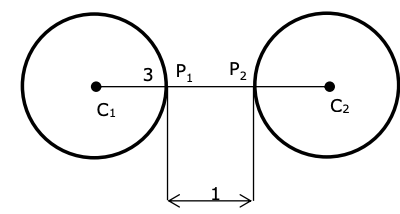So (P1P2)min = 1

Question 25: If (2021)3762 is divided by 17, then the remainder is______

Solution:

(2021)3762 = (2023 – 2)3762 = multiple of 17 + 23762

= 17λ + 22 (24)940

= 17λ + 4 (17 – 1)940

= 17λ + 4 (17μ + 1)

17k + 4; (k ∈ I)

Remainder = 4

Question 26: If [ . ] represents the greatest integer function, then the value of |∫0√π/2 [[x2] - cosx] dx| is ________

Solution:

0√π/2 [[x2] - cosx] dx

= ∫01 [- cosx] dx + ∫1√π/2 [1 - cosx] dx

= ∫01 - 1 - [cosx] dx + ∫1√π/2 dx + ∫1√π/2 - 1 - [cosx] dx

= - ∫01 dx + ∫1√π/2 dx - ∫1√π/2 dx

= - (x)01

= - 1

Question 27: If f (x) = sin [cos-1 (1 - 22x) / (1 + 22x)] and its first derivative with respect to x is (- b / a) loge 2 when x = 1, where a and b are integers, then the minimum value of |a2 - b2| is_______

Solution:

cos-1 [1 - 4x] / [1 + 4x]

Let 2x = t > 0

cos-1 [(1 - t2) / (1 + t2)], t > 0 and t = tan θ

cos-1 (cos 2θ) = 2θ ∈ (0, π), θ ∈ π / 2, 2θ ∈ (0, π)

⇒ 2θ

sin {cos-1 [[1 - 4x] / [1 + 4x]]}

= sin2θ

y = [2 tanθ / (1 + tan2 θ)] = 2t / (1 + t2) = [2 * 2x] / [1 + 4x]

dy / dx = [20 ln 2 - 32 ln 2] / 25 = - 12 ln 2 / 25

a =25, b= 12

|a2 - b2|min = |252 - 122| = 481

Question 28: If the function f (x) = [cos (sinx) - cosx] / x4 is continuous at each point in its domain and f (0) = 1 / k, then k is ___________

Solution:

(1 / k) = limx→0 [{2 sin (sinx + x) / 2} sin {x - sinx} / 2] / x4

= limx→0 [(sinx + x) (x - sinx)] / 2x4 = {limx→0 [sinx + x] / 2x} {limx→0 (x - sinx) / x3}

= 1 * limx→0 [1 - cosx] / 3x2 = 1 / 6

Question 29: The maximum value of z in the following equation z = 6xy + y2, where 3x + 4y ≤ 100 and 4x + 3y ≤ 75 for x ≥ 0 and y ≥ 0 is _________

Solution:

x ≥ 0, y ≥ 0

4x + 3y ≤ 75

3x + 4y ≤ 100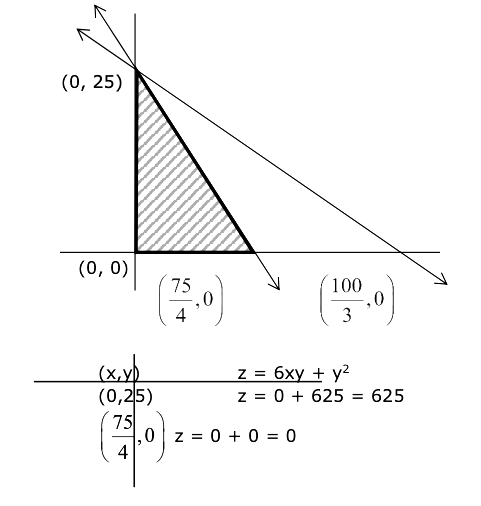(0, 0), z = 0 + 0 = 0

zmax = 625 at x = 0, y = 25

Solution:

### JEE Main 2021 Maths Paper March 17 Shift 1